• 有查询条件就查询，多个查询条件，只要有查询，就增加一个查询条件//类型if($sotype){$where['type']=$sotype;}//合作单位if($companyid){$where['hezuodanwei']=$companyid;}//关键词模糊查询$type是变量if($key){$... 有查询条件就查询， 多个查询条件，只要有查询，就增加一个查询条件 //类型 if($sotype){

$where['type'] =$sotype;

}

//合作单位

if($companyid){$where['hezuodanwei'] = $companyid; } //关键词 模糊查询$type 是变量

if($key){$where[$type] = ['like',"%".$key."%"];

}

$rs=Db::name('student')->where($where)->order('id desc')->limit($limit)->page($page)->select();

$rs1=Db::name('student')->where($where)->select();

$where['type'] =$sotype;

$where['hezuodanwei'] =$companyid;

$where["username"] = ['like',"%".$tag["kw"]."%"];//模糊查询

$where[]=['exp','FIND_IN_SET(2,needID)']; 例子：id in(1,5,8)$where['hezuodanwei'] =array('in','10,12');

组成查询数组$where where($where)

引用：http://blog.csdn.net/u010447573/article/details/47420063

Where 条件表达式格式为：

$map['字段名'] = array('表达式', '操作条件'); 其中$map 是一个普通的数组变量，可以根据自己需求而命名。上述格式中的表达式实际是运算符的意义：

ThinkPHP运算符 与 SQL运算符 对照表

TP运算符SQL运算符例子实际查询条件

eq

=

$map['id'] = array('eq',100); 等效于：$map['id'] = 100;

neq

!=

$map['id'] = array('neq',100); id != 100 gt >$map['id'] = array('gt',100);

id > 100

egt

>=

$map['id'] = array('egt',100); id >= 100 lt <$map['id'] = array('lt',100);

id < 100

elt

<=

$map['id'] = array('elt',100); id <= 100 like like$map = array('like','Admin%');

between

between and

$map['id'] = array('between','1,8'); id BETWEEN 1 AND 8 not between not between and$map['id'] = array('not between','1,8');

id NOT BETWEEN 1 AND 8

in

in

$map['id'] = array('in','1,5,8'); id in(1,5,8) not in not in$map['id'] = array('not in','1,5,8');

id not in(1,5,8)

and(默认)

and

$map['id'] = array(array('gt',1),array('lt',10)); (id > 1) AND (id < 10) or or$map['id'] = array(array('gt',3),array('lt',10), 'or');

(id > 3) OR (id < 10)

xor(异或)

xor

两个输入中只有一个是true时，结果为true，否则为false，例子略。

1 xor 1 = 0

exp

综合表达式

$map['id'] = array('exp','in(1,3,8)');$map['id'] = array('in','1,3,8');

补充说明

同 SQL 一样，ThinkPHP运算符不区分大小写，eq 与 EQ 一样。

between、 in 条件支持字符串或者数组，即下面两种写法是等效的：

$map['id'] = array('not in','1,5,8');$map['id'] = array('not in',array('1','5','8'));

exp 表达式

上表中的 exp 不是一个运算符，而是一个综合表达式以支持更复杂的条件设置。exp 的操作条件不会被当成字符串，可以使用任何 SQL 支持的语法，包括使用函数和字段名称。

exp 不仅用于 where 条件，也可以用于数据更新，如：

$Dao = M("Article"); // 构建 save 的数据数组，文章点击数+1$data['id'] = 10;

$data['counter'] = array('exp','counter+1'); // 根据条件保存修改的数据$User->save($data); 官方查询语法：https://www.kancloud.cn/manual/thinkphp5/135182 查询表达式 版本新增功能 5.0.9 比较运算增加闭包子查询支持 5.0.4 支持对同一个字段多次调用查询方法 查询表达式支持大部分的SQL查询语法，也是ThinkPHP查询语言的精髓，查询表达式的使用格式： where('字段名','表达式','查询条件'); whereOr('字段名','表达式','查询条件'); 表达式不分大小写，支持的查询表达式有下面几种，分别表示的含义是： 表达式含义 EQ、= 等于(=) NEQ、<> 不等于(<>) GT、> 大于(>) EGT、>= 大于等于(>=) LT、< 小于( ELT、<= 小于等于(<=) LIKE 模糊查询 [NOT] BETWEEN (不在)区间查询 [NOT] IN (不在)IN 查询 [NOT] NULL 查询字段是否(不)是NULL [NOT] EXISTS EXISTS查询 EXP 表达式查询，支持SQL语法 > time 时间比较 < time 时间比较 between time 时间比较 notbetween time 时间比较 表达式查询的用法示例如下： EQ ：等于(=) 例如： where('id','eq',100); where('id','=',100); 和下面的查询等效 where('id',100); 表示的查询条件就是 id = 100 NEQ： 不等于(<>) 例如： where('id','neq',100); where('id','<>',100); 表示的查询条件就是 id <> 100 GT：大于(>) 例如： where('id','gt',100); where('id','>',100); 表示的查询条件就是 id > 100 EGT：大于等于(>=) 例如： where('id','egt',100); where('id','>=',100); 表示的查询条件就是 id >= 100 LT：小于( 例如： where('id','lt',100); where('id',' 表示的查询条件就是 id < 100 ELT： 小于等于(<=) 例如： where('id','elt',100); where('id','<=',100); 表示的查询条件就是 id <= 100 [NOT] LIKE： 同sql的LIKE 例如： where('name','like','thinkphp%'); 查询条件就变成 name like 'thinkphp%' V5.0.5+版本开始，like查询支持使用数组 where('name','like',['%think','php%'],'OR'); [NOT] BETWEEN ：同sql的[not] between 查询条件支持字符串或者数组，例如： where('id','between','1,8'); 和下面的等效： where('id','between',[1,8]); 查询条件就变成 id BETWEEN 1 AND 8 [NOT] IN： 同sql的[not] in 查询条件支持字符串或者数组，例如： where('id','not in','1,5,8'); 和下面的等效： where('id','not in',[1,5,8]); 查询条件就变成 id NOT IN (1,5, 8) [NOT] IN查询支持使用闭包方式 [NOT] NULL ： 查询字段是否(不)是Null，例如： where('name', null); where('title','null'); where('name','not null'); 如果你需要查询一个字段的值为字符串null或者not null，应该使用： where('title','=', 'null'); where('name','=', 'not null'); EXP：表达式 支持更复杂的查询情况 例如： where('id','in','1,3,8'); 可以改成： where('id','exp',' IN (1,3,8) '); exp查询的条件不会被当成字符串，所以后面的查询条件可以使用任何SQL支持的语法，包括使用函数和字段名称。 展开全文• 如需有条件地从表中选取数据，可将 WHERE 子句添加到 SELECT 语句中。语法以下是 SQL SELECT 语句使用 WHERE 子句从数据表中读取数据的通用语法：SELECT field1,field2,...fieldN FROM table_name1,table_name2......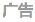2017年9月13日 我们知道从 MySQL 表中使用 SQL SELECT 语句来读取数据。 如需有条件地从表中选取数据，可将 WHERE 子句添加到 SELECT 语句中。 语法 以下是 SQL SELECT 语句使用 WHERE 子句从数据表中读取数据的通用语法：SELECT field1,field2,...fieldN FROM table_name1,table_name2... [WHERE condition1[AND[OR]]condition2.....查询语句中你可以使用一个或者多个表，表之间使用逗号, 分割，并使用WHERE语句来设定查询条件。 你可以在 WHERE 子句中指定任何条件。 你可以使用 AND 或者 OR 指定一个或多个条件。 WHERE 子句也可以运用于 SQL 的 DELETE 或者 UPDATE 命令。 WHERE 子句类似于程序语言中的 if 条件，根据 MySQL 表中的字段值来读取指定的数据。 以下为操作符列表，可用于 WHERE 子句中。 下表中实例假定 A 为 10, B 为 20操作符描述实例 =等号，检测两个值是否相等，如果相等返回true(A = B) 返回false。 <>, !=不等于，检测两个值是否相等，如果不相等返回true(A != B) 返回 true。 >大于号，检测左边的值是否大于右边的值, 如果左边的值大于右边的值返回true(A > B) 返回false。 >=大于等于号，检测左边的值是否大于或等于右边的值, 如果左边的值大于或等于右边的值返回true(A >= B) 返回false。 <=小于等于号，检测左边的值是否小于于或等于右边的值, 如果左边的值小于或等于右边的值返回true(A <= B) 返回 true。 如果我们想再 MySQL 数据表中读取指定的数据，WHERE 子句是非常有用的。 使用主键来作为 WHERE 子句的条件查询是非常快速的。 如果给定的条件在表中没有任何匹配的记录，那么查询不会返回任何数据。 从命令提示符中读取数据 我们将在SQL SELECT语句使用WHERE子句来读取MySQL数据表 chenweiliang_tbl 中的数据： 实例 以下实例将读取 chenweiliang_tbl 表中 chenweiliang_author 字段值为 Sanjay 的所有记录： SQL SELECT WHERE 子句 SELECT*from chenweiliang_tbl WHERE chenweiliang_author=''; MySQL 的 WHERE 子句的字符串比较是不区分大小写的。 你可以使用 BINARY 关键字来设定 WHERE 子句的字符串比较是区分大小写的。 如下实例: BINARY 关键字 mysql> SELECT * from chenweiliang_tbl WHERE BINARY chenweiliang_author='chenweiliang.com'; Empty set (0.01 sec) mysql> SELECT * from chenweiliang_tbl WHERE BINARY chenweiliang_author='chenweiliang.com'; +-----------+---------------+---------------+-----------------+ | chenweiliang_id | chenweiliang_title | chenweiliang_author | submission_date | +-----------+---------------+---------------+-----------------+ | 3 | JAVA 教程 | chenweiliang.com | 2016-05-06 | | 4 | 学习 Python | chenweiliang.com | 2016-03-06 | +-----------+---------------+---------------+-----------------+ 2 rows in set (0.01 sec) 实例中使用了 BINARY 关键字，是区分大小写的，所以 chenweiliang_author='chenweiliang.com' 的查询条件是没有数据的。 使用PHP脚本读取数据 你可以使用 PHP 函数的 mysqli_query() 及相同的 SQL SELECT 带上 WHERE 子句的命令来获取数据。 该函数用于执行 SQL 命令，然后通过 PHP 函数 mysqli_fetch_array() 来输出所有查询的数据。 实例 以下实例将从 chenweiliang_tbl 表中返回使用 chenweiliang_author 字段值为 chenweiliang.com 的记录： MySQL WHERE 子句测试： php$dbhost = 'localhost:3306'; // mysql服务器主机地址

$dbuser = 'root'; // mysql用户名$dbpass = '123456'; // mysql用户名密码

$conn = mysqli_connect($dbhost, $dbuser,$dbpass);

if(! $conn ) { die('连接失败: ' . mysqli_error($conn));

}

// 设置编码，防止中文乱码

mysqli_query($conn , "set names utf8"); // 读取 chenweiliang_author 为 chenweiliang.com 的数据$sql = 'SELECT chenweiliang_id, chenweiliang_title,

chenweiliang_author, submission_date

FROM chenweiliang_tbl

WHERE chenweiliang_author="chenweiliang.com"';

mysqli_select_db( $conn, 'chenweiliang' );$retval = mysqli_query( $conn,$sql );

if(! $retval ) { die('无法读取数据: ' . mysqli_error($conn));

}

echo '

';

echo '

 教程 ID 标题 作者 提交日期

while($row = mysqli_fetch_array($retval, MYSQL_ASSOC))

{

echo "

{$row['chenweiliang_id']} ". " {$row['chenweiliang_title']} ".

"

{$row['chenweiliang_author']} ". " {$row['submission_date']} ".

"

";

}

echo '

';

// 释放内存

mysqli_free_result($retval); mysqli_close($conn);

?>

展开全文• id = 'NZ' AND ( (helpme.category_id = 'M10' AND helpme.band_id = '010') OR (helpme.category_id = 'M10' AND helpme.band_id = '020') ) 在第一类别上工作正常,但是一旦我添加新的WHERE条件失败： SELECT ...

我有一张帮助选择产品的桌子.

我想查询一下我在哪里找回每条记录：

category_id = 'M10' and band_id = '010'

OR

category_id = 'M10' and band_id = '020'

AND

category_id = 'M50' and band_id = '010'

OR

category_id = 'M50' and band_id = '020'

我的查询：

SELECT

product.entry_id AS entry_id,

helpme.item_id AS product_id

FROM inbound_help_me_choose helpme

LEFT JOIN exp_channel_titles product ON (product.product_id = helpme.item_id)

WHERE

helpme.submarket_id = 'NZ'

AND

(

(helpme.category_id = 'M10' AND helpme.band_id = '010')

OR

(helpme.category_id = 'M10' AND helpme.band_id = '020')

)

在第一个类别上工作正常,但是一旦我添加新的WHERE条件失败：

SELECT

product.entry_id AS entry_id,

helpme.item_id AS product_id

FROM inbound_help_me_choose helpme

LEFT JOIN exp_channel_titles product ON (product.product_id = helpme.item_id)

WHERE

helpme.submarket_id = 'NZ'

AND

(

(helpme.category_id = 'M10' AND helpme.band_id = '010')

OR

(helpme.category_id = 'M10' AND helpme.band_id = '020')

)

AND

(

(helpme.category_id = 'M50' AND helpme.band_id = '010')

OR

(helpme.category_id = 'M50' AND helpme.band_id = '020')

OR

(helpme.category_id = 'M50' AND helpme.band_id = '030')

)

对我来说似乎没关系,所以我完全失去了为什么它不起作用.

展开全文• products表如下：a WHERE后以and 接两个条件以及三个条件进行搜索：b 使用or来搜索多个条件c 下面这个有点意思，找出由vend_id 为1002或者1003生产的价格大于10的产品，这里需要使用and 和 or进行组合：但是从上面的...

WHERE可以按多条件进行搜索。

products表如下：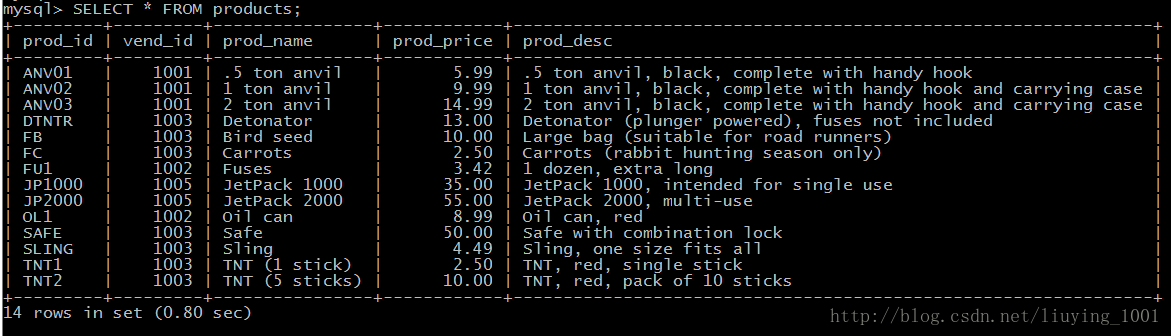a WHERE后以and 接两个条件以及三个条件进行搜索：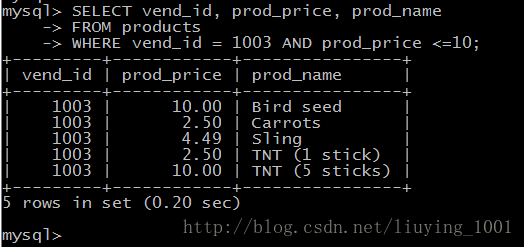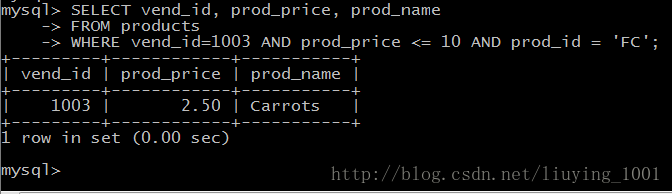b 使用or来搜索多个条件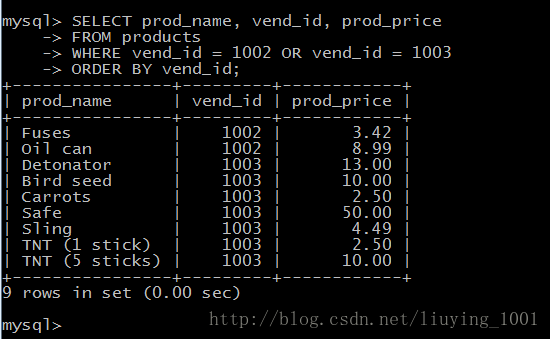c 下面这个有点意思，找出由vend_id 为1002或者1003生产的价格大于10的产品，这里需要使用and 和 or进行组合：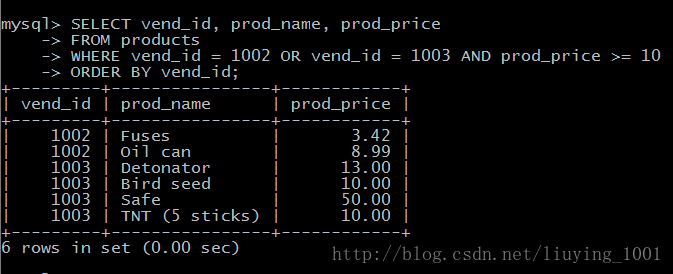但是从上面的结果看，这个输出并不对，原因是AND 比 OR优先级高，因此这个语句的意思变为了，检索出 vend_id=1002的所有产品，以及 vend_id=1003并且价格大于10的产品，所以需要使用()来改写此句：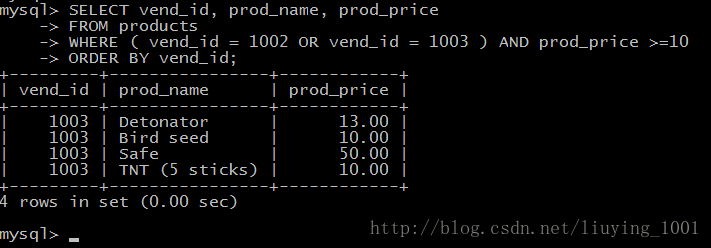d 使用IN 来说明多个搜索值，功能与OR类似，但是有多个值时，使用IN时语法更加直观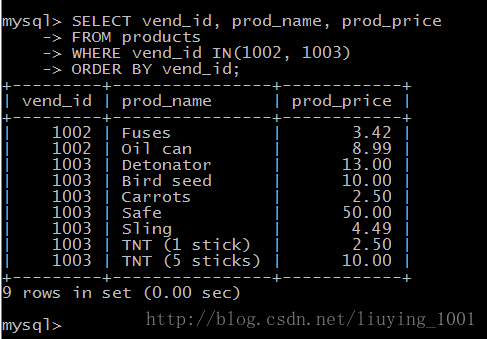e NOT操作符否定其后跟的条件：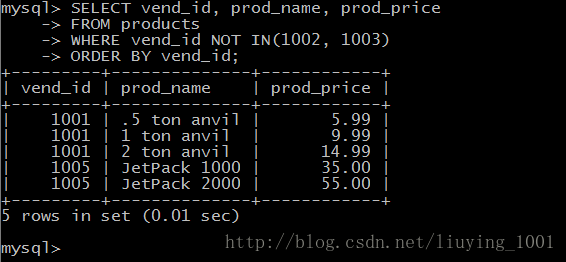在MySQL中，NOT可以用来取反IN、BETWEEN和EXISTS，与其他DBMS系统不同。

原文：http://blog.csdn.net/liuying_1001/article/details/37700883

展开全文• Oracle Where子句

2021-05-01 09:38:13
在本教程中将学习如何使用Oracle WHERE子句来指定过滤的条件返回符合查询条件的行记录。Oracle WHERE子句简介WHERE子句指定SELECT语句返回符合搜索条件的行记录。下面说明了WHERE子句的语法：SELECTcolumn_1,column_...
• SQL的WHERE子句中包含多个AND和OR关于SQL的where子句中包含多个and和or是计算顺序问题，SQL在处理操作时会优先处理 and 操作，这就是很多人会得到很多错误的原因，举个例子：加入有表product字段如下：id、product_...
• 要了解with子句的分组依据，让我们创建一表。创建表的查询如下-mysql>createtableGroupByWithWhereClause->(->IdintNOTNULLAUTO_INCREMENT,->IsDeletedtinyint(1),->MoneyStatusvarchar(20),->...
• 我试图创建一触发器来更新另一个条件检查表，但我不断收到一MySQL错误。我的触发器的代码是：DELIMITER CREATEbTRIGGER visitor_validationBEFORE INSERT ON ratingsvisitorsFOR EACH ROWBEGINSet @counts=...
• 数据库MySQL之where条件查询

千次阅读 2021-02-01 19:28:55
数据库MySQL之where条件查询1. where条件查询的介绍使用where条件查询可以对表中的数据进行筛选，条件成立的记录会出现在结果集中。where语句支持的运算符:比较运算符逻辑运算符模糊查询范围查询空判断where条件查询...
• WHERE 条件 有时候操作数据库时，只操作一些有条件限制的数据，这时可以在SQL语句中添加WHERE子句来规定数据操作的条件。 语法： SELECT column,… FROM tb_name WHERE definition WHERE 关键字后面接有效的表达式...
• WHERE 条件 有时候操作数据库时，只操作一些有条件限制的数据，这时可以在SQL语句中添加WHERE子句来规定数据操作的条件。 语法： SELECT column,… FROM tb_name WHERE definition WHERE 关键字后面接有效的表达式...
• oracle中sql语句中的in的条件数量大于1000有问题oracle中sql语句中select * from t_Test t where t.Id in(1,2,3......)/*数量不能大于1000*/解决方法 分割成次in 然后再或上 如 select * from t_Test t where t....oracle大于条件
• -- where字句-- 删除ID值大于10的用户DELETE FROM register WHERE ID>10;--删除手机号不为...-- 弱语法，这个值可以用数字也可以用字符串-- AND OR:连接多个条件-- 删除id=5 或者手机号=18900000005用户DELE...
• 在使用MySQL SELECT语句时，可以使用 WHERE 子句来指定查询条件，从 FROM 子句的中间结果中选取适当的数据行，达到数据过滤的效果。语法格式如下：WHERE {，，…} 其中，判定运算其结果取值为 TRUE、FALSE 和 ...
• MySql where 查询条件与运算符

千次阅读 2021-01-19 04:25:13
where 是 sql语句 中的条件关键字，类似于其他语言的额 if，只要是条件查询，就一定需要用到各种 条件运算符 来配合，我们就用下面的表来进行简单的学习测试。mysql> select * from test;+----+------+------+| ...
• Py之pandas：利用where、replace等函数对dataframe格式数据按照条件进行数据替换 ... #T2、利用replace()函数：多个数值的字典格式批量替换 #Py之pandas：利用where、replace等函数对datafram...
• 本部分内容主要包括：MySQL中常用的条件查询语句(where)、模糊查询语句(like)、分组语句(group by)、聚合函数(having)、排序语句(order by)。P18 简单查询语句1.查询指定的列的数据：select 列名1,列名2,… From ...
• MySQL数据库 ​ MySQL 数据库是一种广泛使用的关系型数据库，特别是web项目，基本上都是使用 mysql ...​ 作为关系型数据库，MySQL 数据库同样有四核心要素：数据行、数据列、数据表和数据库。同时 mysql 数据库有数据库 mysql sql
• where条件查询

2021-03-26 10:57:55
1. where条件查询的介绍 使用where条件查询可以对表中的数据进行筛选，条件成立的记录会出现在结果集中。 where语句支持的运算符: 比较运算符 逻辑运算符 模糊查询 范围查询 空判断 where条件查询语法格式...mysql 数据库 sql
• exp username/password@192.168.x.x/orcl file = F:\nc\bd_corp.dmp tables="(bd_corp)" query = \"where PK_CORP IN(1015,1156) AND TS < '2017-12-31 23:59:59' AND TS > '2015-01-01 00:00:00' \"另导入...
• mysql中where条件查询

千次阅读 2021-01-18 18:09:34
#进阶2：条件查询/*语法：SELECT查询列表FROM表名WHERE筛选条件；分类：一、按条件表达式筛选条件运算符：> < = <> >= <=二、按逻辑表达式筛选逻辑运算符：作用：用于连接条件表达式&& ||...
• 例如：laravel框架中 很复杂的业务，都需要根据参数判断model的where条件 问题描述： 例如：如果判断条件都是where还好，可以下列写法： $where = []; if($type==1){ \$where['name'] = 'zhangsan'; } ......